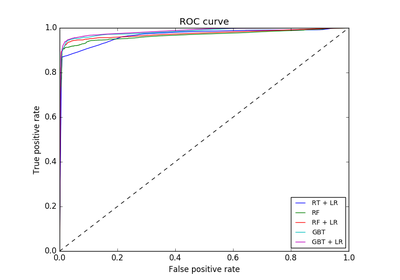# `sklearn.preprocessing`.OneHotEncoder¶

class `sklearn.preprocessing.``OneHotEncoder`(n_values='auto', categorical_features='all', dtype=<type 'numpy.float64'>, sparse=True, handle_unknown='error')[source]

Encode categorical integer features using a one-hot aka one-of-K scheme.

The input to this transformer should be a matrix of integers, denoting the values taken on by categorical (discrete) features. The output will be a sparse matrix where each column corresponds to one possible value of one feature. It is assumed that input features take on values in the range [0, n_values).

This encoding is needed for feeding categorical data to many scikit-learn estimators, notably linear models and SVMs with the standard kernels.

Note: a one-hot encoding of y labels should use a LabelBinarizer instead.

Read more in the User Guide.

Parameters: n_values : ‘auto’, int or array of ints Number of values per feature. ‘auto’ : determine value range from training data. int : number of categorical values per feature.Each feature value should be in `range(n_values)` array : `n_values[i]` is the number of categorical values in`X[:, i]`. Each feature value should be in `range(n_values[i])` categorical_features : “all” or array of indices or mask Specify what features are treated as categorical. ‘all’ (default): All features are treated as categorical. array of indices: Array of categorical feature indices. mask: Array of length n_features and with dtype=bool. Non-categorical features are always stacked to the right of the matrix. dtype : number type, default=np.float Desired dtype of output. sparse : boolean, default=True Will return sparse matrix if set True else will return an array. handle_unknown : str, ‘error’ or ‘ignore’ Whether to raise an error or ignore if a unknown categorical feature is present during transform. active_features_ : array Indices for active features, meaning values that actually occur in the training set. Only available when n_values is `'auto'`. feature_indices_ : array of shape (n_features,) Indices to feature ranges. Feature `i` in the original data is mapped to features from `feature_indices_[i]` to `feature_indices_[i+1]` (and then potentially masked by active_features_ afterwards) n_values_ : array of shape (n_features,) Maximum number of values per feature.

`sklearn.feature_extraction.DictVectorizer`
performs a one-hot encoding of dictionary items (also handles string-valued features).
`sklearn.feature_extraction.FeatureHasher`
performs an approximate one-hot encoding of dictionary items or strings.
`sklearn.preprocessing.LabelBinarizer`
binarizes labels in a one-vs-all fashion.
`sklearn.preprocessing.MultiLabelBinarizer`
transforms between iterable of iterables and a multilabel format, e.g. a (samples x classes) binary matrix indicating the presence of a class label.
`sklearn.preprocessing.LabelEncoder`
encodes labels with values between 0 and n_classes-1.

Examples

Given a dataset with three features and two samples, we let the encoder find the maximum value per feature and transform the data to a binary one-hot encoding.

```>>> from sklearn.preprocessing import OneHotEncoder
>>> enc = OneHotEncoder()
>>> enc.fit([[0, 0, 3], [1, 1, 0], [0, 2, 1], [1, 0, 2]])
OneHotEncoder(categorical_features='all', dtype=<... 'numpy.float64'>,
handle_unknown='error', n_values='auto', sparse=True)
>>> enc.n_values_
array([2, 3, 4])
>>> enc.feature_indices_
array([0, 2, 5, 9])
>>> enc.transform([[0, 1, 1]]).toarray()
array([[ 1.,  0.,  0.,  1.,  0.,  0.,  1.,  0.,  0.]])
```

Methods

 `fit`(X[, y]) Fit OneHotEncoder to X. `fit_transform`(X[, y]) Fit OneHotEncoder to X, then transform X. `get_params`([deep]) Get parameters for this estimator. `set_params`(\*\*params) Set the parameters of this estimator. `transform`(X) Transform X using one-hot encoding.
`__init__`(n_values='auto', categorical_features='all', dtype=<type 'numpy.float64'>, sparse=True, handle_unknown='error')[source]
`fit`(X, y=None)[source]

Fit OneHotEncoder to X.

Parameters: X : array-like, shape [n_samples, n_feature] Input array of type int. self :
`fit_transform`(X, y=None)[source]

Fit OneHotEncoder to X, then transform X.

Equivalent to self.fit(X).transform(X), but more convenient and more efficient. See fit for the parameters, transform for the return value.

`get_params`(deep=True)[source]

Get parameters for this estimator.

Parameters: deep : boolean, optional If True, will return the parameters for this estimator and contained subobjects that are estimators. params : mapping of string to any Parameter names mapped to their values.
`set_params`(**params)[source]

Set the parameters of this estimator.

The method works on simple estimators as well as on nested objects (such as pipelines). The latter have parameters of the form `<component>__<parameter>` so that it’s possible to update each component of a nested object.

Returns: self :
`transform`(X)[source]

Transform X using one-hot encoding.

Parameters: X : array-like, shape [n_samples, n_features] Input array of type int. X_out : sparse matrix if sparse=True else a 2-d array, dtype=int Transformed input.

## Examples using `sklearn.preprocessing.OneHotEncoder`¶Feature transformations with ensembles of trees